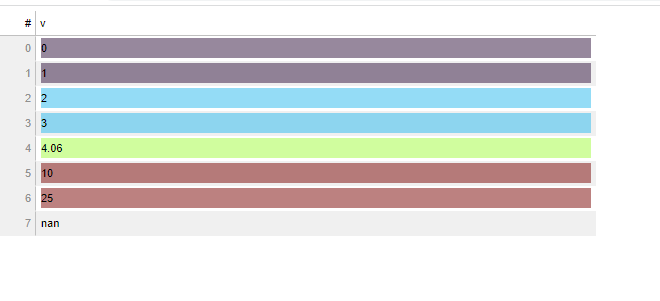# Colormapping on TableColumn

My challenge: essentially port the LinearColorMapper to the background-color of a table.

My solution, while working, is on the janky side. I poached the core logic from the BokehJS source code → bokeh/log_color_mapper.ts at branch-3.2 · bokeh/bokeh · GitHub and used to drive an HTMLTemplateFormatter… i.e. I basically reused the logic and wrote a LinearColorMapper function inside the template:

``````from bokeh.models import HTMLTemplateFormatter, DataTable, TableColumn, ColumnDataSource
from bokeh.plotting import save
from bokeh.palettes import Turbo5

l = 0
h = 10
pl = [x+'80' for x in Turbo5] #adds 50% opacity to each color hex
pl = "['"+"','".join(pl)+"']" #turns list in it's string equiv....

ss = """
style ="background-color: <%=(function f(v){
const pl   = """+pl+"""
const low = """+str(l)+"""
const high = """+str(h)+"""

if (v>=low&&v<=high){
const norm_factor = 1/(high-low)
const norm_interval = 1/pl.length
var p = (v-low)*norm_factor
var i = Math.floor(p/norm_interval)
if (i==pl.length){return pl[pl.length-1]}
else {
return pl[Math.floor(p/norm_interval)]
}
}
else if (v<low){
return pl
}
else if (v>high){
return pl[pl.length-1]
}
else {return '#ffffff00'}
}(value))%>";
"""

def get_html_formatter(style_string):
'''basic template generation that handles rounding numbers if they are numbers'''
template = '<div '+style_string+'''>
<%=
(function fm(v){
if (typeof v == 'number'){
if (v % 1 == 0){
return v.toFixed(0)}
else {return v.toFixed(2)}}
else {return value}
}(value))%>
</div>
'''
return HTMLTemplateFormatter(template=template)

# cmap = LinearColorMapper(low=0,high=5,palette=Turbo256)
src= ColumnDataSource({'v':[0,1,2,3,4.0567,10,25,'nan']})
col = TableColumn(field='v',title='v',formatter=get_html_formatter(ss))
tbl = DataTable(columns=[col],source=src)
save(tbl,r'C:\Repo\Proofing\tableformatting.html')
``````My challenge/question to the community is if there is a better way, ideally one that actually leverages the code already written (i.e. using BokehJS built in stuff). I originally experimented with instantiating a LinearColorMapper in the template via `Bokeh.get('LinearColorMapper')` but ran into a wall trying to use it… tips/help much appreciated!

This topic was automatically closed 90 days after the last reply. New replies are no longer allowed.